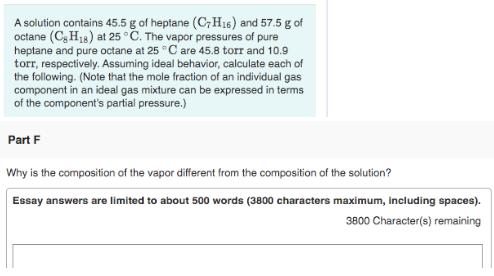# A solution contains 45.5 g of heptane (C7H16) and 57.5 g of octane (C8H18) at 25°C. The vapor pressure of pure heptane and pure octane at 25°C are 45.8 torr and 10.9 torr, respectively. Assuming ideal behavior, calculate each of the following. (Note that the mole fraction of an individual gas component in an ideal gas mixture can be expressed in terms of the component's partial pressure.) Why is the composition of the vapor different from the composition of the solution?